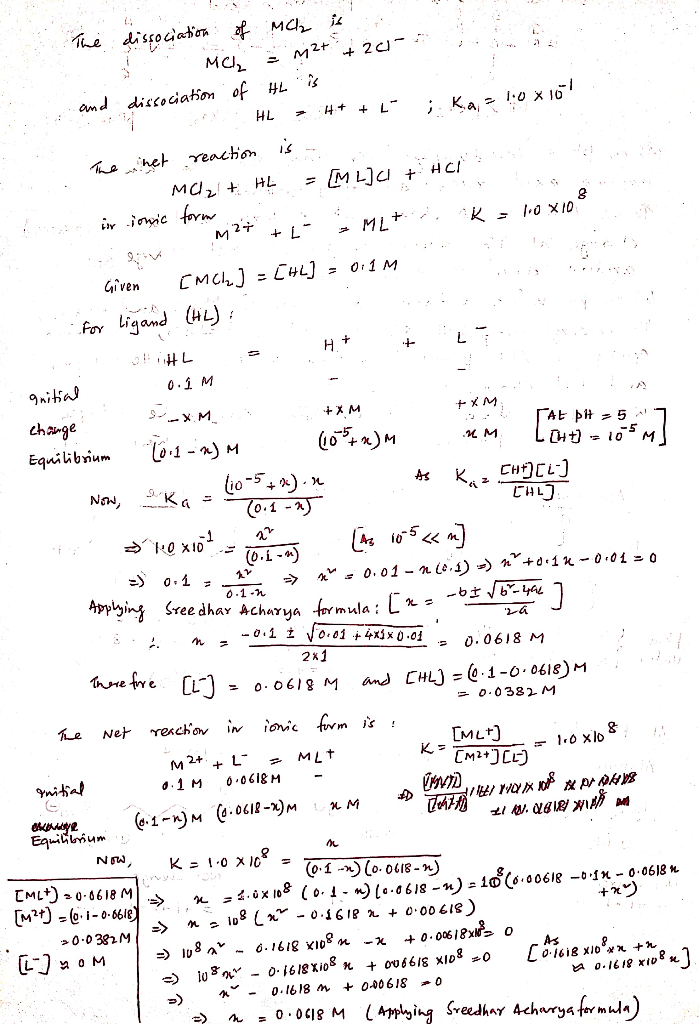Homework Help Question & Answers

A solution is prepared by mixing Mt moles of the salt MCl2 (which dissociates completely to M2+ + 2Cl-) and Lt moles of the ligand HL in 1 L. The following reactions may occur: M2+ + L-= ML+ K= 1.0 *...

A solution is prepared by mixing Mt moles of the salt MCl2 (which dissociates completely to M2+ + 2Cl-) and Lt moles of the ligand HL in 1 L. The following reactions may occur: M2+ + L-= ML+ K= 1.0 * 10^8 HL(aq)=L- +H+ Ka = 1.0*10^-1 write a mass glance for the metal species write a mass balance for ligand species write a charge balance Suppose that Mt=Lt =0.1 M and the pH is somehow fixed at 5.00 use the equilibrium and the two mass balance to find concentration of ML+, M2+, L- and HLAdd Answer of: A solution is prepared by mixing Mt moles of the salt MCl2 (which dissociates completely to M2+ + 2Cl-) and Lt moles of the ligand HL in 1 L. The following reactions may occur: M2+ + L-= ML+ K= 1.0 *...
More Homework Help Questions Additional questions in this topic.

• A brine solution of salt flows at a constant rate of 9 L/min into a large tank that initially held 100 L of brine solution in which was dissolved 0.2 kg of salt. The solution inside the tank is kept...

Need Online Homework Help?

Get FREE EXPERT Answers
WITHIN MINUTES
Related Questions